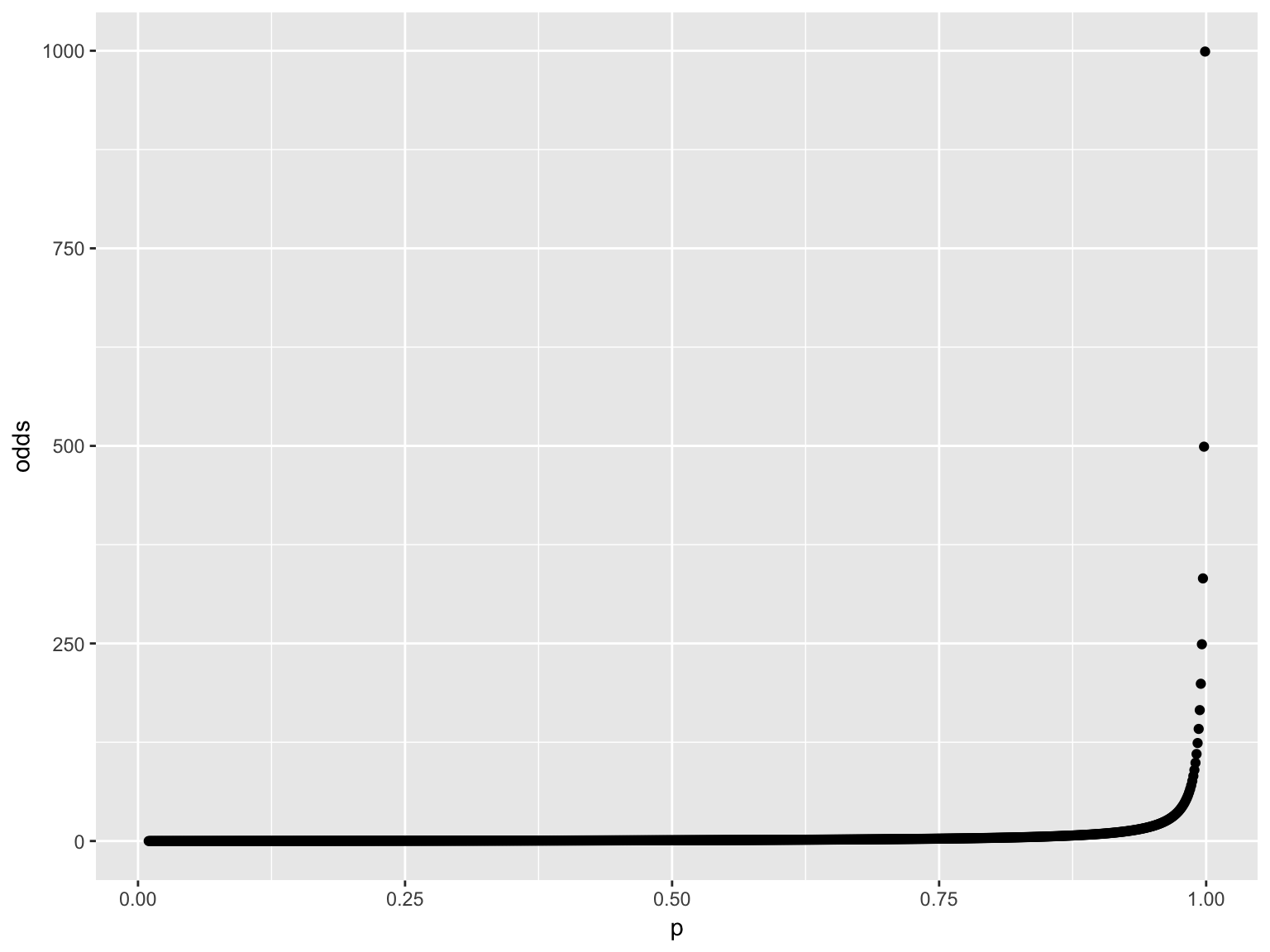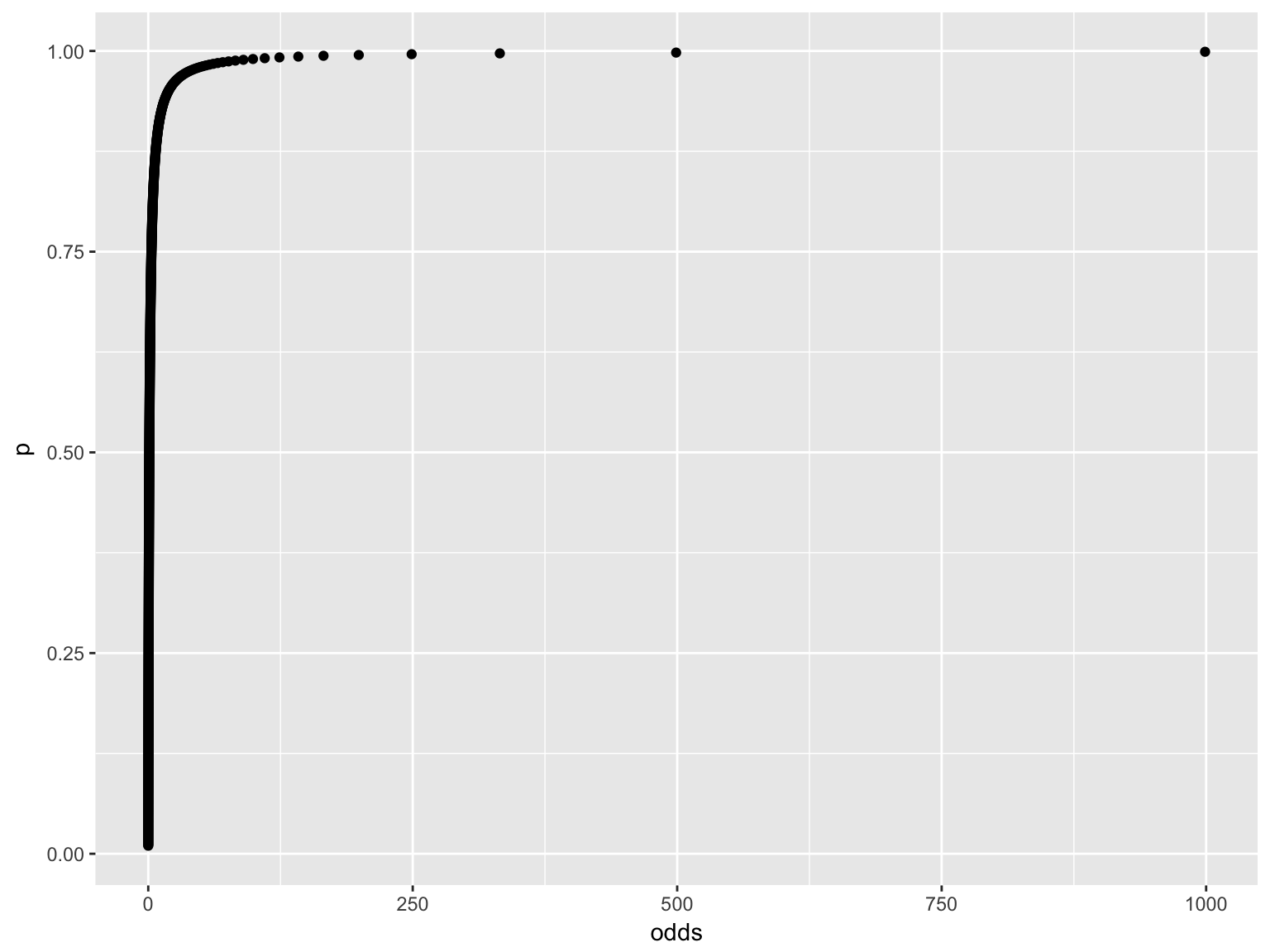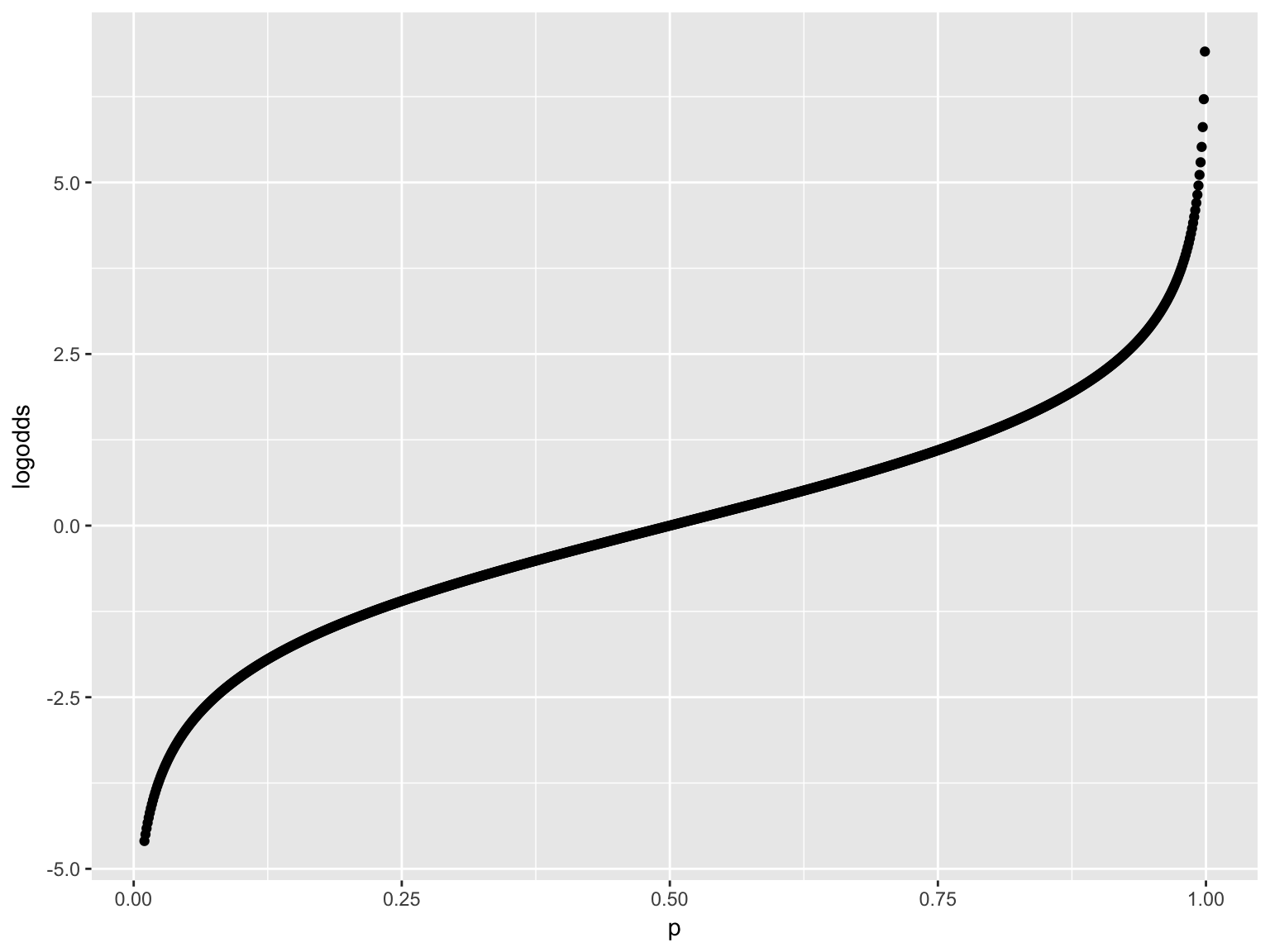# Probability, Odds & Logistic Regression

4/8/2018

library(tidyverse)
## Loading tidyverse: ggplot2
## Loading tidyverse: dplyr
## Conflicts with tidy packages ----------------------------------------------
## filter(): dplyr, stats
## lag():    dplyr, stats

# Probability and Odds

• Two different ways of describing the same thing.

• prababilities are in the range $$0$$ to $$1$$.

• Odds are transformed probabilities

$Odds = \frac{p}{1-p}$

• If $$p = 0$$, $$Odds = 0$$.

• If $$p$$ approaches $$1$$, $$Odds$$ approaches infinity.

• $$Odds$$ has values in the range $$0$$ to $$\inf$$.

• Reversing the relationship above, we get

$p = \frac{Odds}{1+Odds}$

# Graphical View

p = seq(from=.01,to=.999,by=.001)
odds = p/(1-p)
df = data.frame(p,odds)
df$logodds = log(df$odds)

df %>% ggplot(aes(p,odds)) + geom_point()df %>% ggplot(aes(odds,p)) + geom_point()df %>% ggplot(aes(p,logodds)) + geom_point()# Model Relationships.

The linear probability model may be stated as

$p = b_0+b_1*x$

The linear probability can produce probabilities outside the range of zero to one, so we assume the logistic regression model instead.

The logistic regression model places the log of the odds on the left hand side.

$log\left(\frac{p}{1-p}\right)=b_0+b_1*x$

If we solve this equation for the odds expression, we get $\left(\frac{p}{1-p}\right) = e^{b_0+b_1*x}$ The next step is to solve this for the probability. Doing this we get

$p = \frac{e^{b_0+b_1*x}}{1+e^{b_0+b_1*x}}$